# GMAT Math : Calculating the area of a sector

## Example Questions

### Example Question #1 : Calculating The Area Of A Sector

A teacher buys a supersized pizza for his after-school club. The super-pizza has a diameter of 18 inches. If the teacher is able to perfectly cut from the center a 36 degree sector for himself, what is the area of his slice of pizza, rounded to the nearest square inch?

28

24

26

27

25

25

Explanation:

First we calculate the area of the pizza. The area of a circle is defined as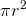. Since our diameter is 18 inches, our radius is 18/2 = 9 inches. So the total area of the pizza is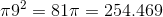square inches.

Since the sector of the pie he cut for himself is 36 degrees, we can set up a ratio to find how much of the pizza he cut for himself. Let x be the area of the pizza he cut for himself. Then we know,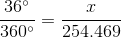Solving for x, we get x=25.45 square inches, which rounds down to 25.

### Example Question #2 : Calculating The Area Of A Sector

In the figure shown below, line segment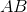passes through the center of the circle and has a length of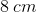. Points, andare on the circle. Sector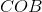covers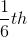of the total area of the circle. Answer the following questions regarding this shape.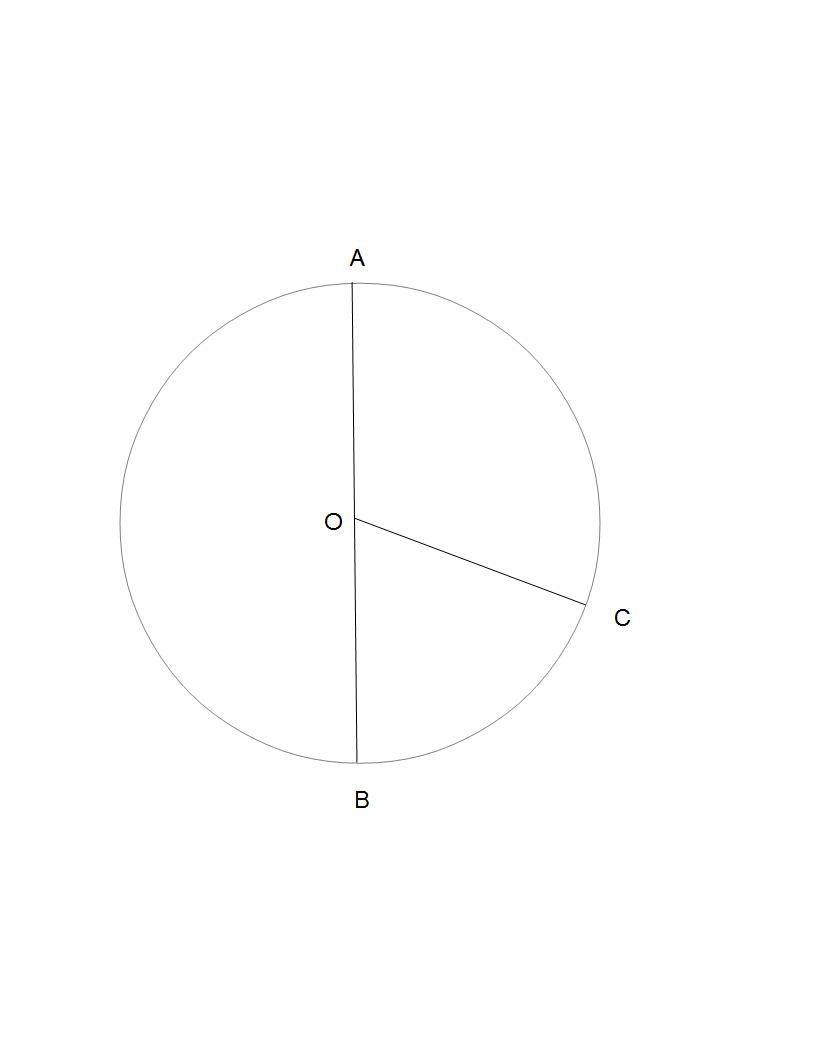Find the area of sector.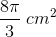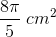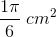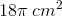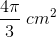Explanation:

To find the area of a sector, we need to know the total area as well as the fractional amount of the sector at which we are looking.

In this case, we find the total area by using the following equation:Because line segmentis our diameter, our radius is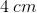. Thus, our total area is: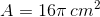We need to go one step further to find the area of sector. Simply multiply the total area by the fractional amount that sectorcovers. We are told it isof the circle's area, so do the following: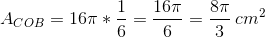Thus, our answer is.

### Example Question #3 : Calculating The Area Of A Sector

Consider the Circle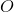: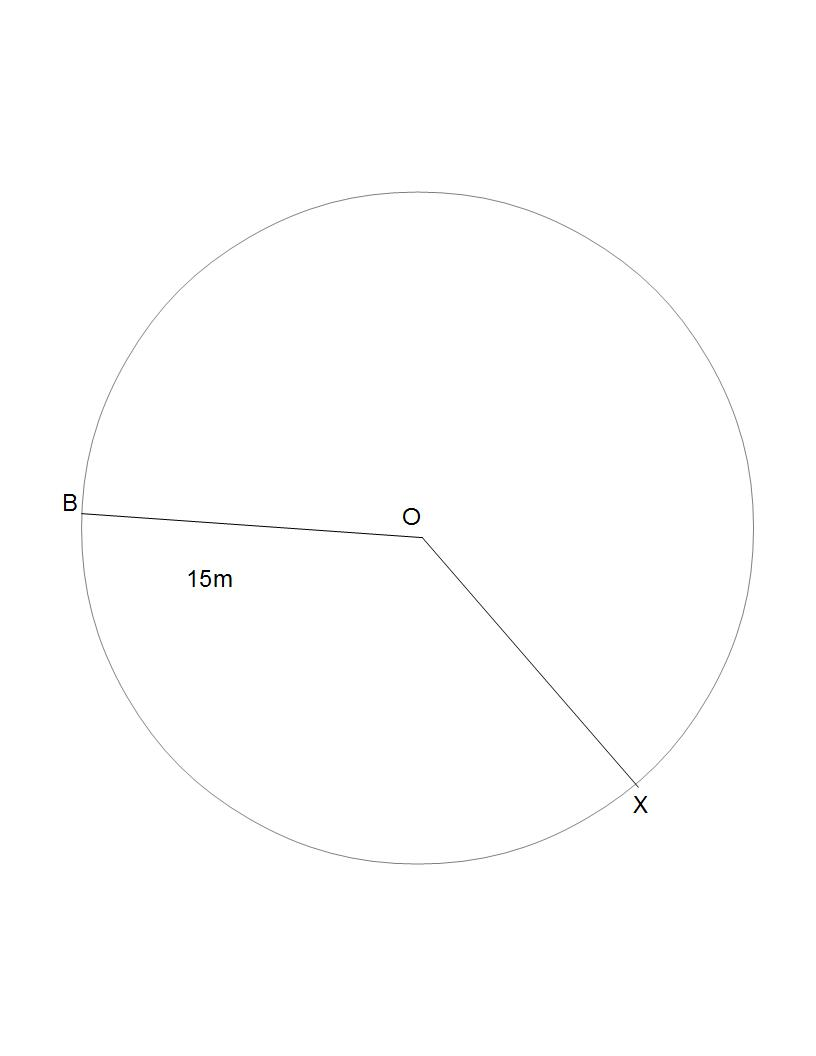(Figure not drawn to scale.)

If angle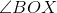is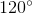, what is the area of sector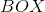in square meters?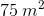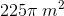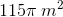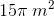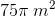Explanation:

To find the area of a sector, simply multiply the total area of the circle by the fraction of the part you are looking at.

In this case, our area will come from the following: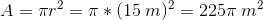To find the fractional part of the circle we care about, take the number of degrees inover the total number of degrees in a circle (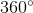):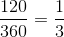So, we find our answer by multiplying these two parts together: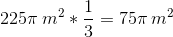### Example Question #4 : Calculating The Area Of A Sector

If a circle has an area of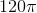, what is the area of a sector with an angle of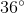?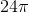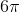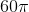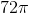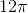Explanation:

The area of a sector with a certain angle will be whatever fraction of the total circle's area the angle of the sector is of. This means we divideby, and then multiply that fraction by the total area of the circle to give us the area of the sector: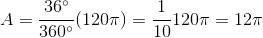### Example Question #5 : Calculating The Area Of A Sector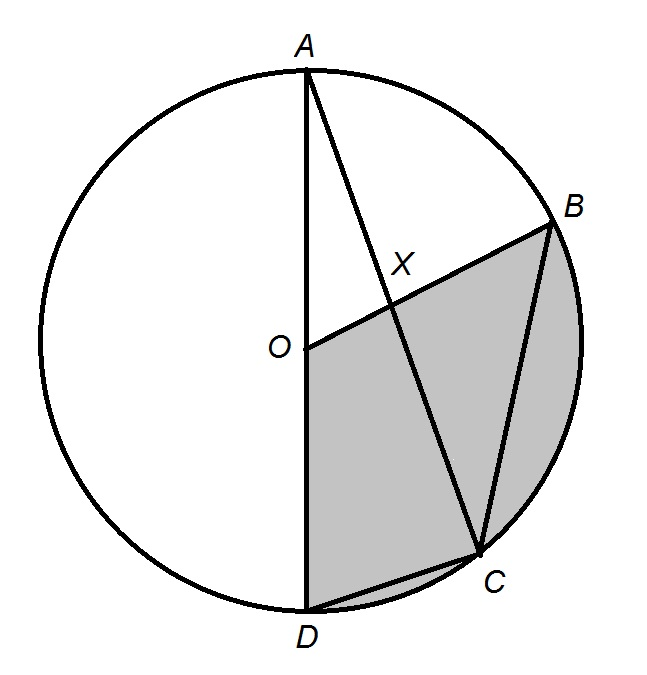The circle in the above diagram has center. Give the area of the shaded sector.

Statement 1: Arc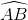has length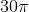.

Statement 2: Arc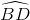has length.

Statement 1 ALONE is sufficient to answer the question, but Statement 2 ALONE is NOT sufficient to answer the question.

BOTH statements TOGETHER are sufficient to answer the question, but NEITHER statement ALONE is sufficient to answer the question.

Statement 2 ALONE is sufficient to answer the question, but Statement 1 ALONE is NOT sufficient to answer the question.

BOTH statements TOGETHER are insufficient to answer the question.

EITHER statement ALONE is sufficient to answer the question.

BOTH statements TOGETHER are sufficient to answer the question, but NEITHER statement ALONE is sufficient to answer the question.

Explanation:

Assume Statement 1 alone. Since the circumference of the circle is not given, it cannot be determined what part of the circleis, and therefore, the central angle of the sector cannot be determined. Also, no information about the circle can be determined. A similar argument can be given for Statement 2 being insufficient.

Now assume both statements are true. Then the length of semicircle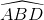is equal to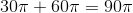. The circumference is twice this, or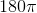. The radius can be calculated as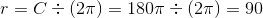, and the area,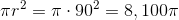. Also,is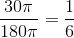of the circle, and the area of the sector can now be calculated as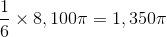.

Tired of practice problems?

Try live online GMAT prep today.